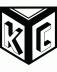## Finding cheaters with k-mers

This semester I'm teaching Perl 6 to beginners. On a recent homework, student A came to see me for help, so I pretty much wrote the script (if you come for help, you get help!). With every assignment, I provide a "test.pl6" script that lets the students know if they will pass. I stress that they don't need to code for edge cases -- just look to pass the test suite. Well, two students, B and C, copied student A, changed a variable name, and submitted.

If I had only checked for passing tests, I wouldn't have noticed, but I like to see how different students try to solve the problems. I'm often pleasantly surprised as a couple of students have some programming background and try to really use the language's strengths. On this occasion, I was disappointed to find that student's B and C (who have little prior coding experience) had turned in my own code.

I thought about how I might automatically detect such cheating because this isn't something a "diff" would catch. We do loads in our lab (Hurwitz Lab, University of Arizona) with k-mers which are "all the possible substrings of length k that are contained in a string" (https://en.wikipedia.org/wiki/K-mer). Just knowing that some number of kmers are shared between any two scripts isn't enough. I need to know if it's significantly more than some baseline which I'll default to the mean of all shared kmers for all pairings of the files in a directory. I then iterate over all combinations and use Student's t-test (https://en.wikipedia.org/wiki/Student%27s_t-test) to find how many standard deviations is the number of shared kmers and report if it's suspiciously high (I default to 5SD, but I found the offenders were >16).

There are loads of things I like about this implementation (apart from the fact that it works). I love being able to get all pair-wise combinations with the "combinations(2) methods of arrays. Bags and Sets make my life so much easier for easy histograms and membership, respectively. I think it's fun to use Unicode characters like in the "std-dev" sub where I square values using a superscript-2.

So, I hope you find this useful. Comments are welcome. I share to help others learn and to learn myself.

```#!/usr/bin/env perl6

subset PosInt of Int where * > 0;

sub MAIN (PosInt :\$k=5, PosInt :\$max-sd=5, *@files) {
die "No files" unless @files;
my @bags = map { find-kmers(+\$k, \$_) }, @files;
my %counts;
for (1..@bags.elems).combinations(2) -> (\$i, \$j) {
my \$bag1  = @bags[\$i-1];
my \$bag2  = @bags[\$j-1];
my \$s1    = \$bag1.Set;
my \$s2    = \$bag2.Set;
my @union = (\$s1 (&) \$s2).keys;
my \$count = (map { \$bag1{ \$_ } }, @union)
+ (map { \$bag2{ \$_ } }, @union);
%counts{"\$i-\$j"} = \$count;
}

my @n  = %counts.values;
my \$mu = mean @n;
my \$sd = std-dev @n;

for %counts.kv -> \$pair, \$kmers {
# https://en.wikipedia.org/wiki/Student%27s_t-test
my \$t = (\$kmers - \$mu) / (\$sd/(@n.elems).sqrt);
if \$t.abs > \$max-sd {
my (\$i, \$j) = \$pair.split('-');
my \$f1 = @files[\$i-1].IO.basename;
my \$f2 = @files[\$j-1].IO.basename;
put "\$pair (\$kmers) = \$t [\$f1, \$f2]";
}
}
}

sub find-kmers (Int \$k, Str \$file) {
# https://en.wikipedia.org/wiki/K-mer
my \$text = \$file.IO.lines.lc.join(' ')
.subst(/:i <-[a..z\s]>/, '', :g).subst(/\s+/, ' ');
\$text.comb.rotor(\$k => -1 * (\$k - 1)).map(*.join).Bag;
}

sub mean (*@n) { @n.sum / @n.elems }

sub std-dev (*@n) {
# https://en.wikipedia.org/wiki/Standard_deviation
my \$mean = mean(@n);
my @dev  = map { (\$_ - \$mean)² }, @n;
my \$var  = @dev.sum / @dev.elems;
return \$var.sqrt;
}
```

Here's what it looked like when I ran it (names changed to protect the innocent):

```\$ ./kmer-counter.pl6 ~/cheaters/*.pl6
6-7 (198) = 16.7509504018028 [s5.pl6, s6.pl6]
2-5 (136) = 5.19428580708723 [s10.pl6, s4.pl6]
1-9 (74) = -6.36237878762838 [s1.pl6, s8.pl6]
5-10 (142) = 6.31267270335003 [s4.pl6, s9.pl6]
3-9 (80) = -5.24399189136558 [s2.pl6, s8.pl6]
2-10 (148) = 7.43105959961283 [s10.pl6, s9.pl6]
4-10 (142) = 6.31267270335003 [s3.pl6, s9.pl6]
7-8 (74) = -6.36237878762838 [s6.pl6, s7.pl6]
3-8 (76) = -5.98958315554078 [s2.pl6, s7.pl6]
6-10 (178) = 13.0229940809268 [s5.pl6, s9.pl6]
4-7 (208) = 18.6149285622408 [s3.pl6, s6.pl6]
4-6 (208) = 18.6149285622408 [s3.pl6, s5.pl6]
8-9 (72) = -6.73517441971598 [s7.pl6, s8.pl6]
2-8 (66) = -7.85356131597878 [s10.pl6, s7.pl6]
1-8 (60) = -8.97194821224158 [s1.pl6, s7.pl6]
5-9 (78) = -5.61678752345318 [s4.pl6, s8.pl6]
5-8 (68) = -7.48076568389118 [s4.pl6, s7.pl6]
\$ ./kmer-counter.pl6 --max-sd=16 ~/cheaters/*.pl6
6-7 (198) = 16.7509504018028 [s5.pl6, s6.pl6]
4-7 (208) = 18.6149285622408 [s3.pl6, s6.pl6]
4-6 (208) = 18.6149285622408 [s3.pl6, s5.pl6]
```I work for Dr. Bonnie Hurwitz at the University of Arizona where I use Perl quite a bit in bioinformatics and metagenomics. I am also trying to write a book at https://www.gitbook.com/book/kyclark/metagenomics/details. Comments welcome.